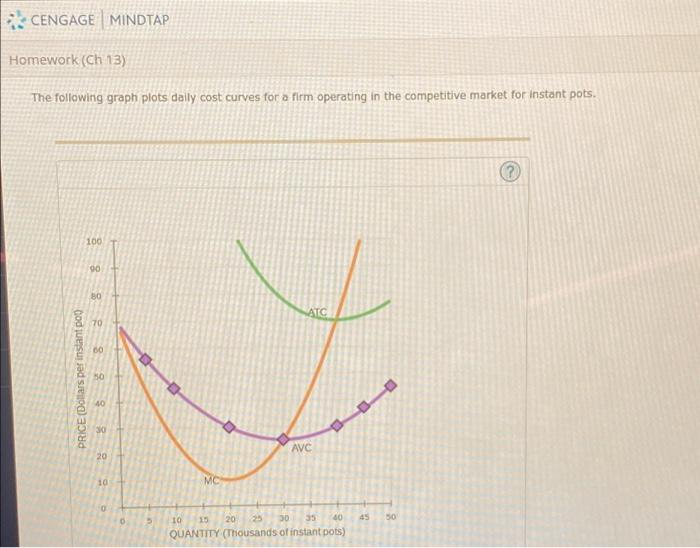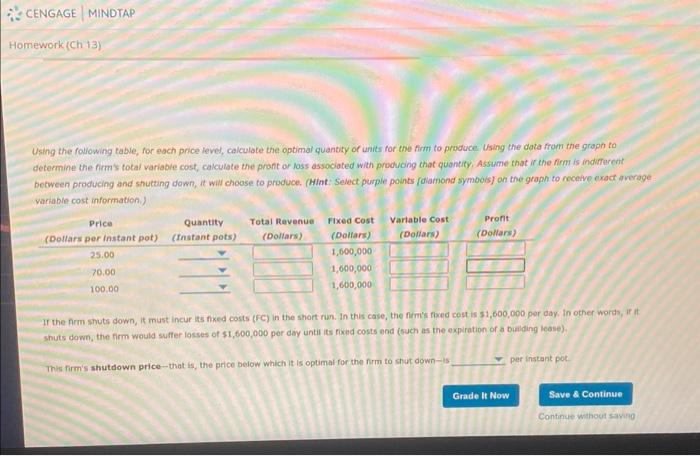Home / Expert Answers / Economics / cengage-mindtap-homework-ch-13-the-following-graph-plots-daily-cost-curves-for-a-firm-operating-in-pa467

# (Solved): CENGAGE MINDTAP Homework (Ch 13) The following graph plots daily cost curves for a firm operating in ...

CENGAGE MINDTAP Homework (Ch 13) The following graph plots daily cost curves for a firm operating in the competitive market for instant pots. PRICE (Dollars per instant pot) 100 90 80 70 60 50 40 30 20 10 0 0 5 MC 10 15 25 30 35 40 QUANTITY (Thousands of instant pots) ATC 20 AVC 45 50 (?)The following graph plots daily cost curves for a firm operating in the competitive market for instant pots. Using the foliowing table, for each price level, calculate the optimal quantity of units for the firm to prowoce Using the dota from the graph to determine the firm ' total variable cost; cakculate the pront or loss associated with producing chat quantity. Assiame that if the firm is indifferent between producing and shutting down, it will choose to produce. (Hint: select purple points folamond symbois) on the graph to noceive cxoct average variable cost information. If the frm shuts down, it must incur its fiked costs (FCC) in the short run. In this case, the firm's fored cost is por day, in other words, if it shuts down, the firm would suffer losses of per dey until its fixad costs end (such as the expiration of a buliding lease) This firm's shutdown price-that is, the price below which it is optimai for the firm to shyt down-is per instant pot.

We have an Answer from Expert

At price 25, output=30000
At price 70, output=40000
At price 100, output=45000
so total revenue at 25=25*30,000=750,000
total revenue at 70=70*40,000=2,800,000
total revenue at 100 =100*45,000=4,500,000

output is where price line intersect the MC curve curve total revenue=price*quantity

We have an Answer from Expert